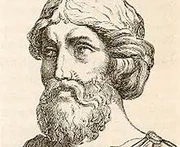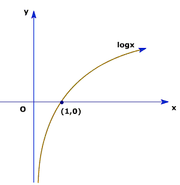## FANDOM

852 PagesThe first mention of prime numbers was by Pythagoras. In October 2013, a new prime number was found.  It is 257,885,161 − 1, also approximately written as 5.818872662322464421751002121132323686363708 × 1017425169 in scientific notation, having a whooping 17,425,170 digits!  This beats the old 2008 record of 243,112,609-1!  It was discovered at the GIMPS (the Great Internet Mersenne Prime Search).  The mathematician Euclid of Alexandria "the Father of Geometry," proved that there is no largest prime number, since there are infinitely large amounts numbers.  Although all mathematicians and geniuses know this, they continue to discover the next one because it is a great hobby.  However, these people could not have done it without the help of some of the most important theorems - two of which is the Prime Numbers Theorem and the Fundamental Theorem of Arithmetic.

## The Prime Number Theorem

The Prime Number Theorem describes how the percentage of numbers that are prime numbers changes as the numbers get higher.  If a number k is randomly chosen from a range of 0 to n, the probabilty that it is prime will approach 1/ln(k) as n approaches infinity.

This means that prime numbers become less common as the sample size increases.

## Mathematical Proof

1.  Let's take the reciprical of all the prime numbers.

That would be 1/2  + 1/3 + 1/5 + 1/7 + ....1/p, where p is the largest prime number.

2.  The divergent series approaches infinity, since there is no limit to this sum.

3.  This shows that there is an infinite number of prime numbers since this sum would only grow until the sum is reached.

### Corollary 1

π(x) is approximately equal to x/(log x-1)The graph of y=log(x). Note how this is similar to the previous graph.

### Corollary 2

The kth prime number is about equal to klog(k).  However, keep in mind that this is still an approximation; there is no real way of determining the exact number.

### Corollary 3

The chance of a random integer k being prime is approximately 1/log(k).  This is true since that log base e of k is equal to ln(e).  Note that their graphs are similar, as shown with the graphs of y=ln (x) and y=log(x) as shown in the above adn right pictures..

## The Fundamental Theorem of Arithmetic

The Fundamental Theorem of Arithmetic, or the unique prime factorization theorem, states that an integer k such that 0<k<∞ is prime itself or composed of other prime numbers (hence the name composite numbers).  Prime factorization is the same for each integer.

For example: the prime factorization of 1000 is 23 x 53.  The factor of primes is always unique, no matter how it is done.  No matter how 1000 is broken up, when expressed as a prime factorization, will be 23 x 53.

## Proof

There are many proofs for this theorem, but three are using the existence theorem, uniqueness theorem, and the proof of the uniqueness theorem.

## The Top Ten Largest Known Prime Numbers

As of 2013, here are the top ten largest known prime numbers!

 Rank Prime number Found by Found date Number of digits 1st 257,885,161 − 1 GIMPS 2013 January 25 17,425,170 2nd 243,112,609 − 1 GIMPS 2008 August 23 12,978,189 3rd 242,643,801 − 1 GIMPS 2009 April 12 12,837,064 4th 237,156,667 − 1 GIMPS 2008 September 6 11,185,272 5th 232,582,657 − 1 GIMPS 2006 September 4 9,808,358 6th 230,402,457 − 1 GIMPS 2005 December 15 9,152,052 7th 225,964,951 − 1 GIMPS 2005 February 18 7,816,230 8th 224,036,583 − 1 GIMPS 2004 May 15 7,235,733 9th 220,996,011 − 1 GIMPS 2003 November 17 6,320,430 10th 213,466,917 − 1 GIMPS 2001 November 14 4,053,946

## Trivia

• Although this might not seem important, the EFF (the Electroninc Frontier Foundation) gives out prizes for new prime numbers discovered in the world.  In 1999, this foundation granted a \$50,000 prize.  This theorem, indirectly. may help mathematicians and other geniuses to find new ones.  Although it is not accurate, it gives a ballpark of numbers rather than shooting at the dark.
• All largest known prime numbers can be able to be expressed in the form 2n-1.
• If the number of digits in the prime number exceeds another certain amount of digits, the foundation will give out another prize.
Community content is available under CC-BY-SA unless otherwise noted.